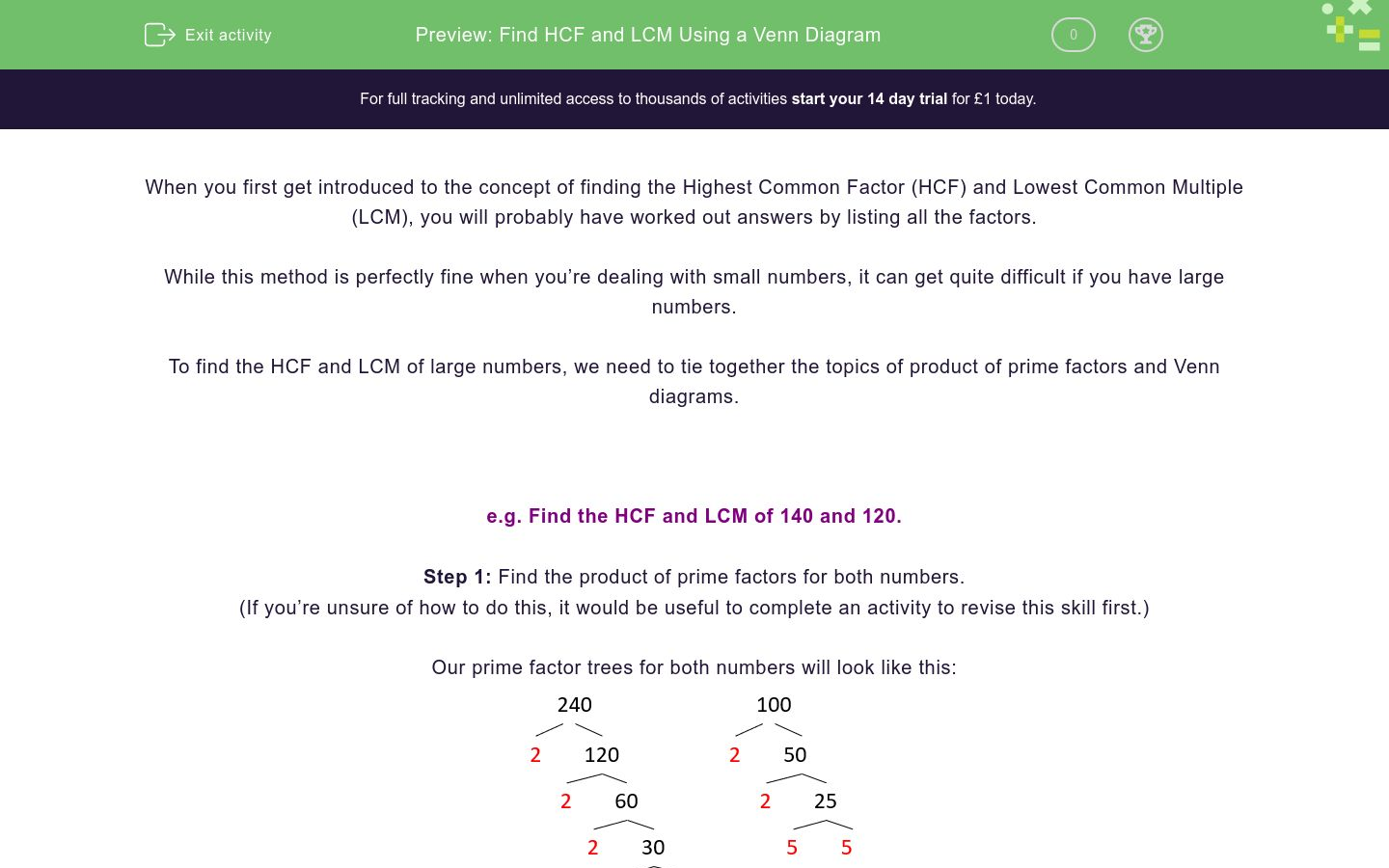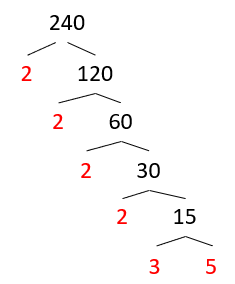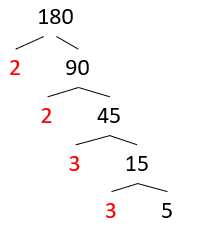# Find HCF and LCM Using a Venn Diagram

In this worksheet, students will practise finding the Highest Common Factors and Lowest Common Multiples of large numbers (with two or three digits), which would be very challenging to calculate using lists.Key stage:  KS 4

GCSE Subjects:   Maths

GCSE Boards:   Pearson Edexcel, OCR, Eduqas, AQA

Curriculum topic:   Number, Number Operations and Integers

Curriculum subtopic:   Structure and Calculation, Whole Number Theory

Difficulty level:### QUESTION 1 of 10

When you first get introduced to the concept of finding the Highest Common Factor (HCF) and Lowest Common Multiple (LCM), you will probably have worked out answers by listing all the factors.

While this method is perfectly fine when you’re dealing with small numbers, it can get quite difficult if you have large numbers.

To find the HCF and LCM of large numbers, we need to tie together the topics of product of prime factors and Venn diagrams.

e.g. Find the HCF and LCM of 140 and 120.

Step 1: Find the product of prime factors for both numbers.

(If you’re unsure of how to do this, it would be useful to complete an activity to revise this skill first.)

Our prime factor trees for both numbers will look like this:Step 2: Identify the numbers which appear in both lists.

240 = 2 x 2 x 2 x 2 x 3 x 5

100 = 2 x 2 x 5 x 5

Step 3: Put these overlapping numbers into the centre of a Venn diagram.

Note that we only put each number in once.Step 4: Put the other numbers from the prime factor trees into the Venn diagram:Step 5: Identify the HCF and LCM.

The HCF can be found by multiplying together all the numbers in the overlap:

2 x 2 x 5 = 20

The LCM can be found by multiplying together all the numbers inside the Venn diagram:

2 x 2 x 3 x 2 x 2 x 5 x 5 = 1200

In this activity, you will find the HCFs and LCMs of larger numbers (with two or three digits) which would be very challenging to calculate using lists.

You will need to have a pen and paper handy so that you can create your own Venn diagrams as you work.

Write 300 as a product of prime factors.

As you should when listing prime common factors, give your answer in ascending order.

Write 240 as a product of prime factors.

What is the HCF of 240 and 300?

2 x 2 x 3 x 5

60

What is the LCM of 240 and 300?

Write 85 as a product of prime factors.

Write 180 as a product of prime factors.

What is the HCF of 85 and 180?

1

5

2

3

What is the LCM of 85 and 180?

Match each number pair below with its HCF.

## Column B

80 and 60
256
150 and 250
20
256 and 1024
50

Match each number pair below with its LCM.

## Column B

80 and 60
240
150 and 250
750
256 and 1024
1024
• Question 1

Write 300 as a product of prime factors.

As you should when listing prime common factors, give your answer in ascending order.

EDDIE SAYS
We need to use a prime factor tree to solve this. Your tree should look like this:Did you remember to write the factors in ascending order? This means smallest to largest.
• Question 2

Write 240 as a product of prime factors.

EDDIE SAYS
Your tree should look like this:You can refer back to the Introduction at any time for an in depth explanation of how to construct and complete these Venn diagrams. Did you remember to list the prime factors in ascending order?
• Question 3

What is the HCF of 240 and 300?

60
EDDIE SAYSThe HCF is the calculated by multiplying the numbers in the overlap together: 2 x 2 x 3 x 5 = 60 Don’t be tempted to leave these as prime factors, if the question asks for the value.
• Question 4

What is the LCM of 240 and 300?

1200
EDDIE SAYSThe LCM is calculated by multiplying all the numbers inside the Venn diagram together: 5 x 2 x 2 x 3 x 5 x 2 x 2 = 1200
• Question 5

Write 85 as a product of prime factors.

EDDIE SAYS
We need to use a prime factor tree to solve this:Did you remember to write the numbers in ascending order, so smallest to largest? This is the order in which prime factors are always recorded unless specifically asked for in another way.
• Question 6

Write 180 as a product of prime factors.

EDDIE SAYS
We need to use a prime factor tree to solve this:We need to make sure they are listed from smallest to largest. For an extra challenge, can you think how to write these prime factors in index form?
• Question 7

What is the HCF of 85 and 180?

5
EDDIE SAYS
If we complete a Venn diagram for these two numbers, it should look something like this:The HCF is calculated by multiplying the numbers in the overlap together. In this case, this is just 5. Again, don’t be tempted to leave answers to this type of question as a list of prime factors or you will lose marks.
• Question 8

What is the LCM of 85 and 180?

3060
EDDIE SAYS
If we complete a Venn diagram for these two numbers, it should look like this:The LCM is simply all the number inside the Venn diagram multiplied together: 17 x 5 x 2 x 2 x 3 x 3 = 3060
• Question 9

Match each number pair below with its HCF.

## Column B

80 and 60
20
150 and 250
50
256 and 1024
256
EDDIE SAYS
Remember the rule for this one: "List the prime factors of both numbers, then the HCF is the greatest number which appears in both lists." e.g. 80 = 1, 2, 4, 5, 8, 10, 16, 20, 40, 80 60 = 1, 2, 3, 4, 5, 6, 10, 12, 15, 20, 30, 60 20 is the highest number which appears in both lists.
• Question 10

Match each number pair below with its LCM.

## Column B

80 and 60
240
150 and 250
750
256 and 1024
1024
EDDIE SAYS
Here's a neat little trick: If you know the HCF (which we found in the last question), you can simply multiply the numbers together and then divide by the HCF, in order to reach the LCM. e.g. The HCF of 80 and 60 is 20. (80 x 60) ÷ 20 = LCM LCM = 240 Otherwise, you can draw Venn diagrams and multiply all the numbers in the diagram together. Amazing work! You may want to practise finding prime common factors if you found this element at all tricky.
---- OR ----

Sign up for a £1 trial so you can track and measure your child's progress on this activity.

### What is EdPlace?

We're your National Curriculum aligned online education content provider helping each child succeed in English, maths and science from year 1 to GCSE. With an EdPlace account you’ll be able to track and measure progress, helping each child achieve their best. We build confidence and attainment by personalising each child’s learning at a level that suits them.

Get started